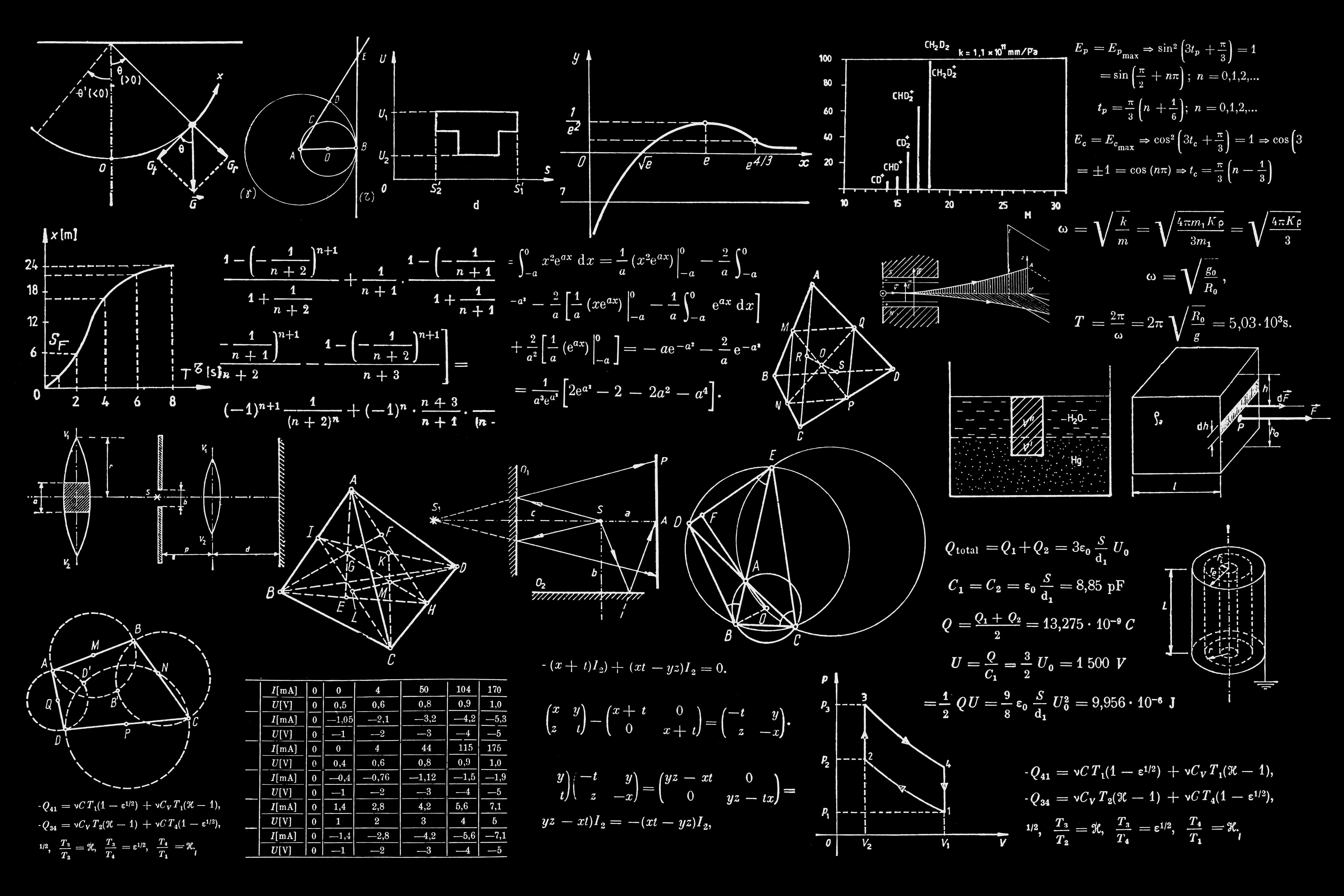## A Step-By-Step Guide to Solving Mathematical Problems in Your Physics CoursePhysics is not an easy subject. And when math is involved in it, it gets even trickier.

So to help you out, here is a step-by-step guide on solving mathematical problems in physics.

#### Know What the Question is Asking For

Before writing the answer, study the question in your physics homework, classwork, assignment, or exam. The goal here is to observe what data your teachers have provided. Understand the overall situation and what they want in the answers.

The question is unlikely to be straightforward. Your teachers will never ask you the answer by simply providing the mathematical data you need to solve them. Instead, they will try to make things tricky. The questions might even have a few twists.

For instance, they will not directly ask you to solve a series circuit. Instead, teachers will talk about how the different elements or nodes in that circuit are connected and then ask you to solve it. They will not mention whether it is a series or parallel circuit. You will have to work that out by yourself. If you are looking for some extra Maths tutoring you should click here.

#### Planning Out the Answer’s Structure

After reading the questions, you can start the answers. You may or may not have solved relevant or similar questions before. It is good if you did solve them, but do not worry if you have not. You can always produce quality answers if you can plan the answer structure.

Carnegie Mellon University did an extensive article stating why students fail to write quality answers. Among the many reasons, failing to communicate the ideas, providing constructive reasoning, and utilizing the provided information are some of the noteworthy points. Even in physics courses, especially when you are providing a mathematical analysis, you need to ensure these qualities in your answers.

If you are confused about how to structure a mathematical answer in physics, refer to the physics answers on a website like sweetstudy.com. These websites provide homework and assignment assistance services to students. Here, the tutors do their research and explain the answers in a structured manner.

Given the thousands of positive reviews these answers get, you can easily rely on their structures. The tutors are professionals and experts in their respective fields. They are helping hundreds of students construct effective answers every day. Hence, you can get a lot of ideas about physics answer structures by going through the solutions these tutors provide.

#### Isolating the Given Data

For solving physics mathematical questions, you need both numeric and theoretical information.

Go through the question again, and try understanding what it is asking for. Then look at the data, and identify the ones you need to solve the problem. Not every numeric value mentioned in the question will be of use to you.

For instance, in calculating the speed of a moving vehicle, you need the values of the distance it travels, and the time it needs for that. You do not need to know the temperature of the air or the length of the vehicle for calculating the speed in an ideal environment. So even if your teacher provides such data, disregard them.

#### Utilizing the Formulas and Doing the Math

You have already isolated the necessary data to solve the problem. Now you just have to use the required formulas to generate the answers.

That is one of the trickiest parts of doing math. A single calculation error can ruin all the hard work. So take your time with this step. Do not rush the answer, and use the calculator carefully. Break down the calculation part into several steps if it helps. You would rather have a correct answer than finish the problem quicker.

#### Working Out the Units

As you put the numeric values in the formula, do the same for the units. So if you are calculating speed, you have to divide the distance by time. The unit of distance is in kilometers and that of time is in hours (for instance). So dividing kilometers by time, you get kilometers per hour, which is the unit of speed.